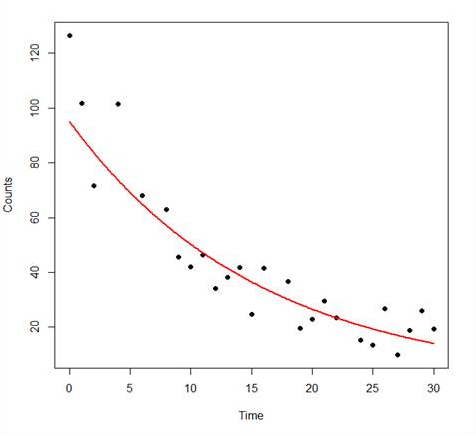# R Is Not So Hard! A Tutorial, Part 5: Fitting an Exponential ModelIn Part 3 and Part 4 we used the lm() command to perform least squares regressions. We saw how to check for non-linearity in our data by fitting polynomial models and checking whether they fit the data better than a linear model. Now let’s see how to fit an exponential model in R.

As before, we will use a data set of counts (atomic disintegration events that take place within a radiation source), taken with a Geiger counter at a nuclear plant.

The counts were registered over a 30 second period for a short-lived, man-made radioactive compound. We read in the data and subtract the background count of 623.4 counts per second in order to obtain` the counts that pertain to the radio-active source.`

Once again, cut and paste the following data into the R workspace.

```A <- structure(list(Time = c(0, 1, 2, 4, 6, 8, 9, 10, 11, 12, 13,
14, 15, 16, 18, 19, 20, 21, 22, 24, 25, 26, 27, 28, 29, 30),
Counts = c(126.6, 101.8, 71.6, 101.6, 68.1, 62.9, 45.5, 41.9,
46.3, 34.1, 38.2, 41.7, 24.7, 41.5, 36.6, 19.6,
22.8, 29.6, 23.5, 15.3, 13.4, 26.8, 9.8, 18.8, 25.9, 19.3)), .Names = c("Time", "Counts"), row.names = c(1L, 2L,
3L, 5L, 7L, 9L, 10L, 11L, 12L, 13L, 14L, 15L, 16L, 17L, 19L, 20L, 21L, 22L, 23L, 25L, 26L, 27L, 28L, 29L, 30L,
31L), class = "data.frame")```

Let’s attach the entire dataset so that we can refer to all variables directly by name.

`attach(A)`
`names(A)`

Let’s see if an exponential function fits the data even better than a quadratic. We set up a grid of points and superpose the exponential function on the previous plot. An exponential function in the Time variable can be treated as a model of the log of the Counts variable.

`exponential.model <- lm(log(Counts)~ Time)`
`summary(exponential.model)`

R returns the following output:

```Call:
lm(formula = log(Counts) ~ Time)

Residuals:
Min       1Q   Median       3Q     Max
-0.54715 -0.17618 0.02855 0.18850 0.55254

Coefficients:
Estimate Std. Error t value Pr(>|t|)
(Intercept) 4.555249   0.111690   40.78 < 2e-16 ***
Time       -0.063915   0.006158 -10.38 2.36e-10 ***
---
Signif. codes: 0 ‘***’ 0.001 ‘**’ 0.01 ‘*’ 0.05 ‘.’ 0.1 ‘ ’ 1

Residual standard error: 0.2814 on 24 degrees of freedom
Multiple R-squared: 0.8178,   Adjusted R-squared: 0.8102
F-statistic: 107.7 on 1 and 24 DF, p-value: 2.362e-10```
Our model predicts the equation as ln(counts) = 4.555249 – 0.063915*time

This model is pretty good, though it explains about 81% of the variance by comparison with the 89% explained by the quadratic model. Let’s plot it on a grid of time values from 0 to 30 in intervals of 0.1 seconds.

`timevalues <- seq(0, 30, 0.1)`
`Counts.exponential2 <- exp(predict(exponential.model,list(Time=timevalues)))`
`plot(Time, Counts,pch=16)`
`lines(timevalues, Counts.exponential2,lwd=2, col = "red", xlab = "Time (s)", ylab = "Counts")`

Note that we used the exponential of the predicted values in the second line of syntax above.So – we have fitted our exponential model. For our data the fitted exponential model fits the data less well than the quadratic model, but still looks like a good model.

In Part 6 we will look at some basic plotting syntax.

About the Author: David Lillis has taught R to many researchers and statisticians. His company, Sigma Statistics and Research Limited, provides both on-line instruction and face-to-face workshops on R, and coding services in R. David holds a doctorate in applied statistics.

1.norman jones says

Your exponential model was made by assuming that the best-fit exponential curve has no vertical or horizontal shift.
If we use a model y=A*exp(k*(t-h))+v

A 24.32223247
k -0.110612853
h 12.99889508
v 14.02693519

this model has a smaller sum of squared differences.

2.ana says

Hi,

Thanks

•Karen Grace-Martin says

Hi Ana,

It’s 4.55 on the log scale. It’s only around 100 once you exponentiate.

3.Abhishek says

Hi ,

I wanted to plot a exponential graph with some data set (like x= cus_id and y=address_id), but how to do it in R serve .

Could you please help me how can i design exponential regression on this data set in R language.

Thanks,
Abhishek

4.desp says

hi,
why you didnt use the nls() instead of lm().Iam asking that because exponential models are non-linear models

5.Alper says

I believe the fitted equation is

log(counts) = 4.555 – log(0.0639)*time

or

counts = e^4.555*(e^-0.0639)^time

or

counts = 95.11*(0.938^time)

•evgen says

it is actually

log(counts) = 4.555 – 0.0639*time

counts = e^(4.555 – 0.0639*time)

counts = e^4.555 * (e^-0.0639*time)

counts = e^4.555 * (e^-0.0639)^time

6.Marina says

Please, would be very helpful if you can confirm that the fitted equation is:

counts = 4.555-log(0.0639)*time

Thank you!

7.Attia says

Could you please write the equation of this fitted curve. Is it

counts = 4.555-log(0.0639)*time

Thanks

8.Conor says

Hi,
How would you increase the slope of the fitted curve?

Please note that, due to the large number of comments submitted, any questions on problems related to a personal study/project will not be answered. We suggest joining Statistically Speaking, where you have access to a private forum and more resources 24/7.